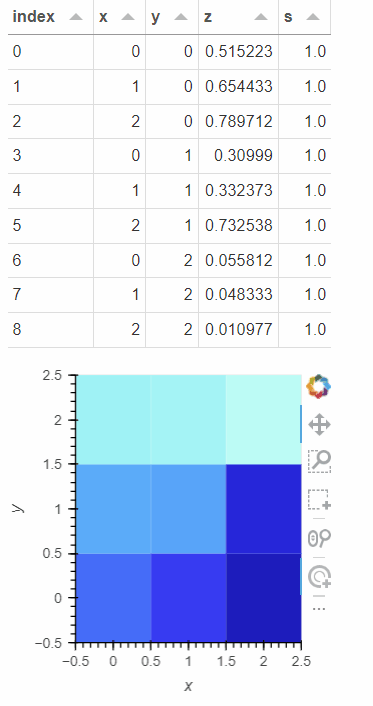# Programmatically Change Selected Data in HeatMap

This is certainly related to can-i-change-which-points-are-selected-programmatically, but the solution there is insufficient.

In my application I generate a heatmap indicating the status of many tasks, with metadata in the tooltips. I want users to be able to click the cell and highlight the corresponding row in a Tabulator widget from Panel. All of this works as expected using `hv.streams.Selection1D`

I also want users to be able to click rows in the table and have the corresponding cells in the heatmap selected - simply the inverse of the above. This what I am struggling to get working. I want to be able to programmatically tell the heatmap what cells have been selected, and get the same alpha applied to the unselected data.

Below is what I have tried so far as MRE, but I am not sure how to get this to work as needed. In addition to what is below, I have also tried updating the `selection_stream`, but this does not propagate the selection back to the heatmap, only to the table.

I am hoping there is an easy way to do this, as I feel like I have overcomplicated everything. Any help is much appreciated!

``````import holoviews as hv
import numpy as np
import pandas as pd
import panel as pn

hv.extension('bokeh')
pn.extension('tabulator')

# setup data
dim = 3
bounds=(-0.5, dim-0.5)
xx, yy = np.meshgrid(range(dim), range(dim))
df = pd.DataFrame({'x': xx.flatten(), 'y': yy.flatten(), 'z': np.random.uniform(size=dim*dim)})

# setup table and heatmap
tb = pn.widgets.Tabulator(df, selectable=True, disabled=True)
heatmap = hv.HeatMap(df, kdims=['x', 'y'], vdims=['z']).opts(tools=['tap', 'box_select'], aspect=1, responsive=True, xlim=bounds, ylim=bounds).redim.range(z=(0, 1.0))

# setup selection stream
selection_stream = hv.streams.Selection1D(source=heatmap)

# callback to update the table selection. need to transpose index
def update_table(index):
tb.selection = [(i % dim) * dim + i // dim for i in index]
return tb

# callback to update the heatmap. this is where the problems are
def update_heatmap(index):
# if no index, return entire heatmap
if not index:
return heatmap
# return a slice of the heatmap using the given indices
# problems:
# - need to reset the bounds and z-range
# - rendered cells are too large until their row/column forces them into a normal size
# - unselected cells simply are not shown, since we filtered them out
# - cannot deselect cells
return heatmap.iloc[index].opts(xlim=bounds, ylim=bounds).redim.range(z=(0, 1.0))

# create simple layout with binds
pn.Column(
pn.bind(update_table, selection_stream.param.index),
pn.bind(update_heatmap, tb.param.selection)
).servable()
``````

I tried but could not get it working. I’ve opened a feature request here.

1 Like

I appreciate you trying to figure it out, and for opening the feature request! I ended working around it by basically just embedding another column in my data to indicate if it was selected, and then used that column as a `dim` assigned to `alpha` parameter. There is a bit of rearranging what I had, but it is not too bad. Having a better built-in solution would certainly be great though!

``````import holoviews as hv
import numpy as np
import pandas as pd
import panel as pn

hv.extension('bokeh')
pn.extension('tabulator')

# setup data
dim = 3
bounds=(-0.5, dim-0.5)
xx, yy = np.meshgrid(range(dim), range(dim))

# added the column 's' to use as the selection to manipulate the alpha
df = pd.DataFrame({'x': xx.flatten(), 'y': yy.flatten(), 'z': np.random.uniform(size=dim*dim), 's': np.ones(shape=dim*dim)})

# callback to update the table selection. need to transpose index
def create_heatmap(data):
if data:
df.loc[data, 's'] = 1.0
df.loc[df.index.difference(data), 's'] = 0.2
else:
df.loc[:, 's'] = 1.0
heatmap = hv.HeatMap(
df,
kdims=['x', 'y'],
vdims=['z', 's']).opts(
tools=['tap', 'box_select'],
aspect=1,
responsive=True,
xlim=bounds,
ylim=bounds,
alpha=hv.dim('s')
).redim.range(z=(0, 1.0))
return heatmap

# update callback
def update_selection(*events):
for event in events:
if event.name == 'index':
# transpose
indices = [(i % dim) * dim + i // dim for i in event.new]
tb.selection = indices
# short circuit to avoid infinite recursion since the line
# right above will retrigger this method
return
pipe.send(event.new)

# setup table
tb = pn.widgets.Tabulator(df, selectable=True, disabled=True)

# create pipe, and use a stream for the heatmap dynamic map,
# and use the dmap as the source for the Selection1D
pipe = hv.streams.Pipe(data=[])
dmap = hv.DynamicMap(create_heatmap, streams=[pipe])
selection_stream = hv.streams.Selection1D(source=dmap)

# set up watchers to trigger the selection update
tb_watcher = tb.param.watch(update_selection, ['selection'], onlychanged=True)
heatmap_watcher = selection_stream.param.watch(update_selection, ['index'], onlychanged=True)

pn.Column(
tb,
dmap
).servable()
``````
1 Like

Example of the above code working as expected1 Like

Congrats on finding a good workaround. And thanks for sharing with the community.